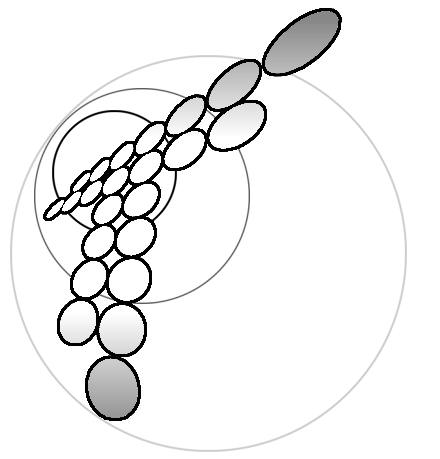# Mathematical modelling of a lead/acid cell with immobilized electrolyte

## Abstract

A mathematical model has been developed for a lead/acid cell with immobilized electrolyte, using the transport equations for concentrated electrolyte solutions and experimentally determined kinetic equations for the two porous electrodes. The model equations have been solved by means of an algorithm, which gives, after discretization of the differential equations, a single tridiagonal coefficient matrix for the concentration profile over the whole multi-region system consisting of a positive electrode, a separator and a negative electrode, respectively. The model can be used for optimization of the actual type of lead/acid cell for different applications. The numerically predicted results are in fair agreement with experimental data for acid and lead sulfate concentration profiles in a cell, although the experimentally measured acid concentration in the negative electrode after discharge is generally lower than the calculated value, and the predicted discharge time is lower than the experimental ones at high discharge rates.

Keywords:Lead/acid cells; Sulfuric acid; Immobilized electrolytes

Skin: